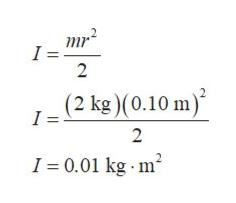# a top with radius 10cm, mass 2 kg has a string around the edge and is initially at rest. assume the top can be reasonably approximated as a solid cylinder. the top is held in place as the string is pulled. the string applies a force of 10N tangential to the edge for 2s.what is the angular velocity (in radians per second) of the top after 3 seconds?what is the angular momentum of the top after the string is pulled?after the string is pulled, the top is released. it travels away from its initial position at a linear velocity of 10m/s. what is the rotational velocity (in radians per s) at this top? assume no friction

Question
18 views

a top with radius 10cm, mass 2 kg has a string around the edge and is initially at rest. assume the top can be reasonably approximated as a solid cylinder. the top is held in place as the string is pulled. the string applies a force of 10N tangential to the edge for 2s.

what is the angular velocity (in radians per second) of the top after 3 seconds?

what is the angular momentum of the top after the string is pulled?

after the string is pulled, the top is released. it travels away from its initial position at a linear velocity of 10m/s. what is the rotational velocity (in radians per s) at this top? assume no friction

check_circle

Step 1

Consider the figure and observe that, force by the string acts tangentially. Therefore, the angle between tangential force and radius of the cylindrical top is 90o.

Step 2

The torque due to the string on the top is computed as follows.

Step 3

As the top is told to be approximated as a solid cylinder. Therefore, ...help_outlineImage Transcriptionclosemr I = 2 (2 kg )(0.10 m) I = 2 I 0.01 kg m fullscreen

### Want to see the full answer?

See Solution

#### Want to see this answer and more?

Solutions are written by subject experts who are available 24/7. Questions are typically answered within 1 hour.*

See Solution
*Response times may vary by subject and question.
Tagged in

### Physics